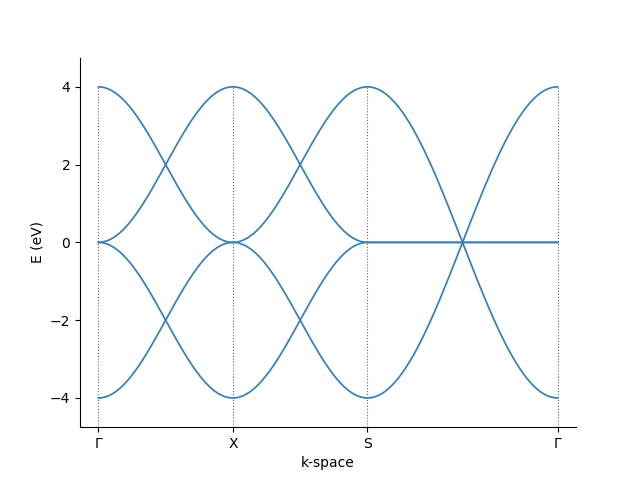# 2. Lattice

Note

A python script that generates KITE's input file requires few python packages that can be included with the following aliases

import kite
import pybinding as pb
import numpy as np
import matplotlib.pyplot as plt


We will first construct a pb.Lattice using Pybinding and calculate its density of states (DOS) using pb.Solver from Pybinding.

Info

If you are familiar with Pybinding, you can go directly to the next tutorial page.

### Making a pb.Lattice¶

The pb.Lattice class from Pybinding carries the information about the TB model. This includes

Pybinding also provides additional functionalities based on this real-space information. It can provide, for example, the reciprocal vectors and the Brillouin zone.

#### Defining the unit cell¶

As a simple example, let us construct a square lattice with a single lattice site. The following syntax can be used to define the primitive lattice vectors:

 1 2 3 4 a1 = np.array([1, 0]) # [nm] defines the first lattice vector a2 = np.array([0, 1]) # [nm] defines the second lattice vector lat = pb.Lattice(a1=a1, a2=a2) # defines a lattice object 

We than add the desired lattice sites inside the unit cell (the same syntax can be used to add different orbitals in a given position or more sites in different sublattices):

 1 2 3 4 5 6 onsite = 0 # onsite potential lat.add_sublattices( # generates a lattice site (sublattice) with a tuple # (name, position, and onsite potential) ('A', [0, 0], onsite) ) 

By default, the main unit cell has the index [n1,n2] = [0, 0]. The hoppings between neighboring sites can be added with the simple syntax:

 1 2 3 4 5 6 lat.add_hoppings( # generated a hopping between lattice sites with a tuple # (relative unit cell index, site from, site to, hopping energy) ([1, 0], 'A', 'A', - 1 ), ([0, 1], 'A', 'A', - 1 ) ) 

Here, the relative indices n1,n2 represent the number of integer steps - in units of the primitive lattice vectors - needed to reach a neighboring cell starting from the origin.

If the lattice has more than one sublattice, the hoppings can connect sites in the same unit cell.

Note

When adding the hopping (n, m) between sites n and m, the conjugate hopping term (m, n) is added automatically. Pybinding does not allow the user to add them by hand.

#### Visualization¶

Now we can plot the pb.lattice and visualize the Brillouin zone:

 1 2 3 4 5 lat.plot() plt.show() lat.plot_brillouin_zone() plt.show() 

Examples

For a crystal with two atoms per unit cell, look in the Examples section. For other examples and pre-defined lattices consult the Pybinding documentation.

### Using Pybinding's solver¶

Pybinding has build-in solvers for

• LAPACK (exact diagonalization) and
• ARPACK (targeted diagonalization of sparse matrices). To use any of these solvers, we need to first construct a model.

#### Building a pb.Model¶

The pb.Model class contains all the information of the structure we want to use in our calculation. This structure can be larger than the unit cell (*stored in the pb.Lattice-class). It can also have specific geometries and other possible modifications of the original lattice. Here, we will just double the unit cell in both directions in the pb.Model and add periodic boundary conditions:

 1 2 3 4 5 model = pb.Model( lat, # pb.Lattice, uses the previously defined unit-cell pb.primitive(2, 2), # doubles the unit-cell in both directions pb.translational_symmetry(a1=2, a2=2) # periodic boundary conditions with period '2' ) 
We can visualise this pb.Model with
 1 2 model.plot() plt.show() 

#### Defining a pb.Solver¶

The pb.Solver class takes a pb.Model class as input and prepares the system to perform a numerical calculation. We will use the LAPACK solver:

 1 2 3 solver = pb.solver.lapack( model # pb.Model, use the previously defined system ) 

#### Band structure calculation¶

As an example, the band structure is calculated using the pb.Solver defined above.

First, for a two-dimensional plot, we must define a path in the reciprocal space that connects the high symmetry points. Using the pb.Lattice build-in method, the high symmetry points for the corners of a path can be found easily:

 1 2 3 4 bz = lat.brillouin_zone() gamma = np.array([0, 0]) x = (bz + bz) / 2 s = bz 

Then, we just pass these corners to the pb.Solver and visualize the result

 1 2 3 4 5 6 7 bands = solver.calc_bands(gamma, x, s, gamma, step=0.01) bands.plot(point_labels=[r"$\Gamma$", "X", "S", r"$\Gamma$"]) plt.show() lat.plot_brillouin_zone(decorate=False) bands.k_path.plot(point_labels=[r"$\Gamma$", "X", "S", r"$\Gamma$"]) plt.show()The visualization of the band structure and its path in the reciprocal space.

  1 2 3 4 5 6 7 8 9 10 11 12 13 14 15 16 17 18 19 20 21 22 23 24 25 26 27 28 29 30 31 32 33 34 35 36 37 38 39 40 41 42 43 44 45 46 47 import kite import pybinding as pb import numpy as np import matplotlib.pyplot as plt a1 = np.array([1, 0]) # [nm] define the first lattice vector a2 = np.array([0, 1]) # [nm] define the second lattice vector lat = pb.Lattice(a1=a1, a2=a2) # define a lattice object onsite = 0 # onsite potential lat.add_sublattices( # make a lattice site (sublattice) with a tuple # (name, position, and onsite potential) ('A', [0, 0], onsite) ) lat.add_hoppings( # make an hopping between lattice site with a tuple # (relative unit cell index, site from, site to, hopping energy) ([1, 0], 'A', 'A', - 1 ), ([0, 1], 'A', 'A', - 1 ) ) model = pb.Model( lat, # pb.Lattice, use the previously defined unit-cell pb.primitive(2, 2), # double the unit-cell in both directions pb.translational_symmetry(a1=2, a2=2) # periodic boundary conditions with period '2' ) solver = pb.solver.lapack( model # pb.Model, use the previously defined system ) bz = lat.brillouin_zone() gamma = np.array([0, 0]) x = (bz + bz) / 2 s = bz bands = solver.calc_bands(gamma, x, s, gamma, step=0.01) bands.plot(point_labels=[r"$\Gamma$", "X", "S", r"$\Gamma$"]) plt.show() lat.plot_brillouin_zone(decorate=False) bands.k_path.plot(point_labels=[r"$\Gamma$", "X", "S", r"$\Gamma$"]) plt.show()